reasoning (43) Maths (40) Home (8) integration (6)

## Mix reasoning practice set in english ,SSC Reasoning Practice Set PDF Download

Mix reasoning practice set in English, SSC Previous Year Reasoning Questions PDF,  Most important  Reasoning  questions with answers  which  includes circle  problems, box problems, triangles problems  for competitive exams like SSC CGL , SSC CHSL ,CPO ,Bank exams and RRB NTPC etc have been explained in this post.

# Ten Important Reasoning questions with answers for competitive exams

## Problem # 1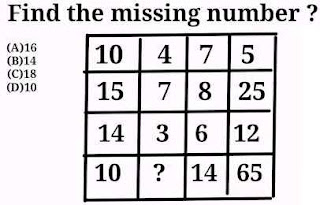This box problem consist of four rows and four columns . And we have to find the value of question mark  in 4th row after studying the pattern of all the numbers in this box. This question mark is in 4th row of 2nd column.
Formula :- (R2 - R3) × R1 = R4

### Calculation

Column 1
(15 -14 ) × 10 = 10
Column 3
(8 - 6 ) × 7 = 14
Column 4
(25 -12 ) × 5 = 65
Column 2
(7 - 3 ) × 4 = 16
Option (A)16 is correct option.

##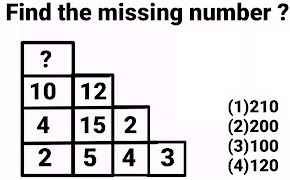This problem figure consists of four rows and every rows from top to bottom have one more row in comparison to previous row.
If we take the product of all the numbers in each row and compare the result so obtained ,then we have same result in every row.

Product of all the numbers in every row is 120.

2 × 5 × 4 × 3 = 120 ( Last Row )
4 × 15 × 2 = 120  (2nd Last Row )
10 × 12 = 120  (2nd   Row )
Hence the value of   "?"   must be 20
Option (4)120 is correct option.

##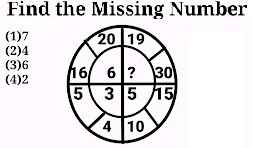This circle consists of four quadrants and every  quadrant consists of three numbers . And every quadrant have two numbers in outer part and one  number  in the inner part . To find the value of question mark  "?"  , we shall use two numbers which are in the outer part to calculate the value of the number which is in the inner part of every quadrant .

Since the question mark is in 1st quadrant ,so we have to start the calculation from 2nd quadrant.

Step 1.   Take sum of both the numbers (16 and 20 ) in outer part of  this quadrant.

Step 2. Take the square root of  the number obtained in step 1.

### Calculation

√( 20 +16) = √(36) =6

Step 1.   Take sum of both the numbers (5 and 4 ) in outer part of  this quadrant.

Step 2. Take the square root of  the number obtained in step 1.

### Calculation

√( 5 + 4 ) = √( 9) =3

Step 1.   Take sum of both the numbers (5 and 4 ) in outer part of  this quadrant.

Step 2. Take the square root of  the number obtained in step 1.

### Calculation

√(15 +10) = √(25) =5

Step 1.   Take sum of both the numbers (5 and 4 ) in outer part of  this quadrant.

Step 2. Take the square root of  the number obtained in step 1.

### Calculation

√( 30 +19) = √(49) = 7
Option  (1)7 is correct option.

##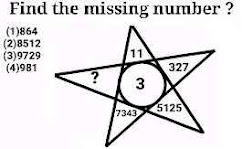Writing the 1st Number as it is and then place its cube on the right side of it. Now moving clockwise pick the next odd number for next position.
1³ = 1  ⇒ 11
3³ = 27 ⇒327
5³ = 125 ⇒5125
7³ = 343 ⇒7343
9³ = 729 ⇒9729

##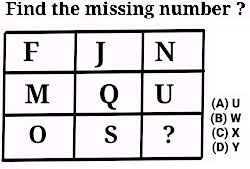This box problem consists of three rows and three columns. To find the value of question marks in 3rd column of 3rd row, we have to add 1st ,2nd and 3th columns since since in every column is same in every row.

Formula :-  .
F + 4 = J  ,  J  + 4 = N
M + 4 = Q , Q + 4 = U
O + 4 = S  ,  S  + 4 = W
Option (B)W is correct option.
This box problem consists of three rows and three columns. To find the value of question marks in 3rd column of 3rd row, we have to add 1st ,2nd and 3th columns since since in every column is same in every row.

Formula :- The sum of 1st three column in every row is same .
B + 1 = D ,  D + 1  = F
N + 1 = P ,  P + 1  = R
Starting from 4th quadrant in anti clockwise direction ,take the sum of  both the numbers in outer part of the quadrant .Then reverse the orders of both the digits of the number so obtained to get the value of the number in the inner part of same quadrant.

9 × 8 = 72 ⟺ 27 (After reversing the orders of digits)

8 × 3 = 24 ⟺ 42 (After reversing the orders of digits)

5 × 7 = 35 ⟺ 53 (After reversing the orders of digits)

7 × 7 = 49 ⟺ 94 (After reversing the orders of digits) (The value of question mark).

Hence option (2)94  is correct.

## Problem # 8

Splitting all the numbers in the given circle into two series

## Any Term = Preceding Term + 4

### 1st term = 28

2nd term = 1st term + 4
2nd term  28  + 4 = 32
2nd term  = 32

3rd term = 2nd term + 4
3rd term  32  + 4 = 36
3rd term   = 36

4th term = 3rd term + 4
4th term 36  + 4 = 40
4th term = 40

## 2nd Series

?  , 31 , 33, 35 with a difference of 2 , So the 1st term of this series must be 2 less than the 2nd term.

## Any Term = Preceding Term - 2

4th term = 5th term - 2
4th term =  37 - 2
4th term = 35

3rd term = 4th term - 2
3rd term =  35 - 2
3rd term = 33

2nd term = 3rd term - 2
2nd term =  33 - 2
2nd term = 31

Therefore 1st term = 2nd term - 2
1st term =  31 - 2
1st term = 29

## Problem # 9

Every term can be expressed with the help of preceding term.

(2 × 1) + 2 = 2 + 2 = 4 ( 2nd number in series)
(4 × 2) - 3 = 8 - 3 = 5 ( 3rd number in series)
(5 × 3) + 4 = 15 + 4 = 19 ( 4th number in series)
(19 × 4) - 5 = 76 - 5  = 71 ( 5th number in series)
(71 × 5) - 6 = 355 - 6  = 349 ( 6th number in series)

## Problem # 10

5 = 1st term
(5 × 3) + 1 = 15 + 1 =16 = 2nd term
(16 × 3) + 2 = 48 + 2 =50 = 3rd term
(50 × 3) + 3 = 150 + 3 =153 = 4th term = value of question mark
(153 × 3) + 4 = 459 + 4 =463 = 5th term
(463 × 3) + 5 = 1389 + 5 = 1394 = 2nd term

Share:

## Box Reasoning questions with answers for competitive exams

Most important Box Reasoning questions with answers which includes circle problems, box problems, circle problems, triangles problems for competitive exams like SSC CGL ,SSC CHSL ,CPO ,Bank exams and RRB NTPC etc have been explained in this post.

# Ten Important Box Reasoning questions with answers for competitive exams

## Problem #1

This reasoning problem consists of three figures and every figure have three numbers associated to it . So the solution of this problem is to find the value of question mark using other two numbers associated to it .

But the main problem is how to utilised these two numbers to get the value of this question mark?
Now watch carefully the 1st two figures . since these figures have some values at bottom lines.
Now we have to find or search the  formula for these two numbers in each figure to utilised them in any possible way to get   number in bottom line.
The same formula will be applicable to third figure to find out the value of question mark.

### Formula :-

The difference of  squares of  greater number to smaller number in upper line in every figure is equal to number in lower line in every figure.

4³  - 6² = 64 - 36 = 28 (1st figure)

3³ - 2² = 27 - 4 = 23 ( 2nd figure)

5³  - 5² = 125 - 25 = 100 (3rd figure), This number will be the value of question mark.

Option (3)100  is correct option

## Problem #2

This reasoning problem also consists of three figures and every figure have three numbers associated to it . So the solution of this problem is to find the value of question mark using other two numbers associated to it .

But the main purpose is how to utilised other two numbers to get the value of this question mark?
Now watch carefully the 1st two figures . since these figures have some values at bottom lines.
Now we have to find or search the  formula for these two numbers in each figure to utilised them in any possible way to get   number in bottom line.
The same formula will be applicable to third figure to find out the value of question mark.

### Formula :-

The sum of cubes of  1st number and  squares  of  2nd number in upper line in every figure is equal to number in lower row in every figure.

4³ + 6² = 64 + 36 = 100 (1st figure)

2³ + 7² = 8 + 49 = 57 (2nd figure)

4³ + 4² = 64 + 16 = 80 (3rd figure)

Option (4)80  is correct option

## Problem #3

### Formula :-

Four times the difference of the 1st columns and 2nd column = Number in 3rd  column.

C3 = 4  ×  (C1 - C2)

4 × ( 9 - 4 ) = 4 × 5 = 20 ( Number in 1st row 3rd column ) .

4 × ( 8 - 5 ) = 4 × 4 = 16 ( Number in 2nd row 3rd column )

4 × ( 7 - 6 ) = 4 × 1 = 2 ( Number in 1st row 3rd column ) .
Option (A)2 is correct option

## Problem #4

This reasoning problem is a box problem of 4 rows and 3 columns, And we have to find the the value of question mark in 3rd column of 3rd row .

### Formula :-

In every column the sum of 1st and 3rd row is equal to sum of 2nd and 4th row.

i.e.  R1 + R3 = R2 + R4

## 1st Column

9 + 7 = 5 + 11 = 12 ( Equal sum in 1st column of R1 & R3 and R2 &R4 )

## 2nd Column

6 + 4 = 8 + 2 = 10 ( Equal sum in 2nd column of R1 & R3 and R2 &R4 )

## 3rd Column

8 + ? = 4 + 7 = 11 ( Equal sum in 3rd column of R1 & R3 and R2 &R4 )

Hence  8 + ?  = 11
? = 11 - 8
? = 3
Option (D)3 is correct option

## Problem #5

This reasoning problem is a box problem of 4 rows and 3 columns, And we have to find the the value of question mark in 3rd column of 2nd row .

### Formula :-

Divide 1st row with the difference of 4th and 3rd row to get the value of 2nd row.

R1  ÷ ( R4 - R3 ) = R2

## 1st Column

25 ÷ ( 20 - 15 ) = 25  ÷ 5 = 5 ( Number in 2nd row 1st  column )

## 2nd Column

49 ÷ ( 20 - 13 ) = 49 ÷ 7 = 7 ( Number in 2nd row 2nd  column )

## 3rd Column

81 ÷ ( 20 - 11 ) = 81 ÷ 9 = 5 = ?  ( Number in 2nd row 3rd column )
Option (B)9 is correct option

## Problem #6

This reasoning problem is a box problem of 4 rows and 3 columns, And we have to find the the value of question mark in 2nd column of 2nd row .

### Formula :-

Add square root of 1st three row to get the value of number in fourth row.

R1  +  R2 + R3  = R4

## 1st Column

√169 + √64 + √81 = 13 + 8 + 9 = 30 ( Number in 4th row of 1st column )

## 3rd Column

√1296 + √576 + √100 = 36 + 24 + 10 = 70  ( Number in 4th row of 3rd column )

## 2nd Column

√625 +  √? + √49 = 25 + √? + 7 =  50

32 + √? = 50

√? = 50 - 32

√? = 18 , Squaring both sides

? = 324 ( Number in 4th row of 2nd column )

Option (A)324  is correct option

## Problem #7

This reasoning problem is also a box problem of 3 rows and 3 columns, And we have to find the the value of question mark in 3rd column of 2nd row .

### Formula :-

Divide the  sum of square of 1st  row to 3rd row to get the value of number in 2nd row.

(R1²  +  R3 ) ÷  2  =  R2.

## 1st Column

( 4² + 4 ) ÷ 2 = ( 16 + 4 ) ÷ 2 = 20 ÷ 2 = 10 ( Number in 2nd row of 1st column )

## 2nd Column

( 18² + 6 ) ÷ 2 = ( 324 + 6 ) ÷ 2 = 330 ÷ 2 =  165 ( Number in 2nd row of  2nd column )

## 3rd Column

( 23² + 1 ) ÷ 2 = ( 529 + 1 ) ÷ 2 = 530 ÷ 2 = 265 ( Number in 2nd row of 3rd column )
Option (B)265  is correct option

## Problem #8

This reasoning problem is a box problem of 4 rows and 3 columns, And we have to find the the value of question mark in 2nd column of 2nd row .

### Formula :-

Add square root of 1st three row to get the value of number in fourth row.

R1  +  R2 + R3  = R4.

## 1st column

2² + 20 = 4 + 20 = 24

## 2nd column

3² + 30 = 9 + 30 = 39

## 3rd column

4² + 40 = 16 + 40 = 56
Option (D)56 is correct option

## Problem #9

In this reasoning problem two clues are given to find the value of question mark. In 1st clue two numbers 27 and 4 (both are on left hand side ) are associated to give result 139(the number of the right hand side) by using any mathematical operations or combination of mathematical operations. And  in 2nd clue two numbers 31 and 9 are associated to give result 319 by using any mathematical operations or combination of mathematical operations. Similarly we have to associate 21 and 6 by using same mathematical operation to find the value of question mark.

### Formula :-

(1st number  × 2nd number ) + (1st number  + 2nd number ) = Number of right hand side

(27 × 4 ) + (27 + 4 ) = 108 + 31 = 139.
(31 × 9 ) + (31 + 9 ) =
279 + 40  = 319.
(21 × 6 ) + (21 + 6 ) = 126 + 27 = 153 = The value of Question mark.
Option (1)153 is correct option

## Problem #10

In this reasoning problem two clues are given to find the value of question mark. In 1st clue two numbers 27 and 4 (both are on left hand side ) are associated to give result 77(the number of the right hand side) by using any mathematical operations or combination of mathematical operations. And  in 2nd clue two numbers 31 and 9 are associated to give result 239 by using any mathematical operations or combination of mathematical operations. Similarly we have to associate 21 and 6 by using same mathematical operation to find the value of question mark.

### Formula :-

(1st number  × 2nd number ) - (1st number + 2nd number )  = Number of right hand side

(27 × 4 ) - (27 + 4 ) = 108 - 31 = 77.
(31 × 9 ) - (31 + 9 ) =
279 - 40 = 239.
(21 × 6 ) - (21 + 6 ) = 126 - 27 = 99 = The value of Question mark.
Option (2)99 is correct option

Share:

## Ten Missing term in box reasoning questions with answers

Ten Missing term in box reasoning , Reasoning questions with answer, box reasoning with solutions have been discussed in this post . These types of problems are very helpful for cracking competitive exams like ssc cgl, ssc chsl , RRB NTPC, Group D and various Bank exams and many other similar exams.

## Problem # 1

Product of both the digits in 1st column is equal to sum of both the digits in 2nd column in every box.

### 1st Box

5 × 2 = 5 + 5 = 10
3 × 2 = 5 + 1 = 6
4 × 3 = 8 + 4 =12

##Sum of squares of both the digits in 1st column is equal to  the Number in 2nd column in every box.

### 1st Box

2² + 1² = 4 + 1 = 5
5² + 4² = 25 + 16 = 41
1² + 1² = 1 + 1 = 2

### 2nd Box

4² + 6² = 16 + 36 = 52
6² + 6² = 36 + 36 = 72
8² +  0² = 64 + 0 = 64

### 3rd Box

2² + 3² = 4 + 9 = 13
2² + 2² = 4 + 4 = 8
1² + 5² = 1 + 25 = 26
Hence correct option is (1)72, 13

## Problem # 3

### Formula :-

Add one to both the digits in 1st column separately in every box to get
the number in 2nd column in every box.

### 1st Box

2 + 1 = 3(Digit in ten's place) , 1 + 1 = 2(Digit in unit's place)
Hence Number in 2nd column will be 32.

2 + 1 = 3(Digit in ten's place) , 2 + 1 = 7(Digit in unit's place)
Hence Number in 2nd column will be 37.

2 + 1 = 3(Digit in ten's place) , 9 + 1 = 10(Digit in unit's place)
Hence Number in 2nd column will be 310

##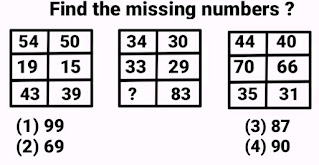### 1st Box

54 - 50 = 4 (1st row)
19 - 15 = 4 (2nd row)
43 - 39 = 4 (3rd row).

### 3rd Box

44 - 40 = 4 (1st row)
70 - 66 = 4 (2nd row)
35 - 31 = 4 (3rd row)

### 2nd Box

34 - 30 = 4 (1st row)
33 - 29 = 4 (2nd row)
? - 83 = 4 (3rd row).
? = 4 + 83
? = 87(The value of question mark).
Hence correct option is (3)87.

##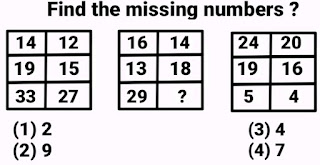### 1st Row

14 + 12 + 16 + 14 + 24 +20 = 100

### 2nd Row

19 + 15 + 13 + 18 + 19 +16 = 100

### 3rd Row

33 + 27 + 29 + ? + 5 +4 = 100
89 + ? + 9 = 100
?  + 98 = 100
? = 100 - 98
? = 2(The value of question mark)
Hence correct option is (1)2.

## Problem # 7

### Formula :-

Product of both the digits in 1st column is equal to sum of both the digits in 2nd column in every box.

### 1st Box

5 × 2 = 5 + 5 = 10
3 × 2 = 5 + 1 = 6
4 × 3 = 8 + 4 = 12

### Formula :-

The product of 1st and 3rd numbers in each column of every box is equal to the Number in middle number in opposite box.

### 1st Box

5 × 7 = 35 Number in middle row opposite to 2nd column (1st box 2nd column )

3 × 5 = 15 Number in middle row opposite to 1st column (1st box 1st column).

### 2nd Box

4 × 2 = 8 Number in middle row opposite to 2nd column (2nd box 2nd column ).

5 × 9 = 45 Number in middle row opposite to 2nd column ( 2nd box 1st column ).

### 3rd Box

7 × 6 = 42 Number in middle row opposite to 2nd column (3rd box 2nd column ).

8× 9 = 72 Number in middle row opposite to 2nd column ( 3rd box 1st column).
Hence correct option is (3)42.

## Problem # 9

### Formula :-

Number in middle row opposite to 2nd column

### 1st Box

5 + 7 = 12 Number in middle row opposite to 2nd column (1st box 2nd column )

3 + 5 = 8 Number in middle row opposite to 1st column (1st box 1st column).

2nd Box

4 + 2 = 6 Number in middle row opposite to 2nd column (2nd box 2nd column).

5 + 9 = 14 Number in middle row opposite to 1st column (2nd box 1st column).

3rd Box

7 + 6 = 13 Number in middle row opposite to 2nd column (3rd box 2nd column) .

8 + 9 = 17 Number in middle row opposite to 1st column (3rd box 1st column).

Hence correct option is (2)17.

## Problem # 10

Formula :-

Product of both the digits in 1st column is equal to product of both the digits in 2nd column in  every row and in every box .

1st Box

5 × 4 = 4 × 5  = 20(1st row 1st box).

1 × 2 = 2 × 1  = 2(2nd row 1st box).

4 × 3 = 2 × 6  = 12(3rd row 1st box).

### 2nd Box

3 × 4 = 6 × 2 = 12(1st row 2nd box).

3 × 3 = 1 × 9 = 9(2nd row 2nd box).

4 × 6 =  8 × 3 = 24(3rd row 2nd box).

### 3rd Box

4 × 4 = 8 × 2 = 16(1st row 3rd box).

6 × 4 = ? × ? = 24(2nd row 3rd box).

So out of four option only the product of both the digits in 4th option is equal to 24.

3 × 5 = 5 × 3 = 15(3rd row 3rd box).

Hence correct option is (4)38

Ten Missing term in box reasoning questions with answers box with solutions discussed in this post . These types of problems are very helpful for cracking competitive exams like ssc cgl, ssc chsl and various Bank exams and many other similar exams. please feel free to comment your opinions regarding this post.

Share:

## Types of matrix with definition , Different types of Matrix discussed in this post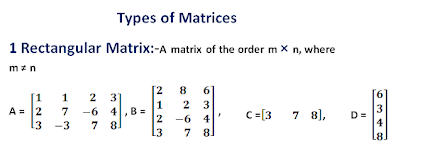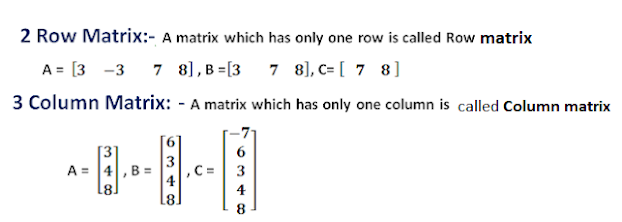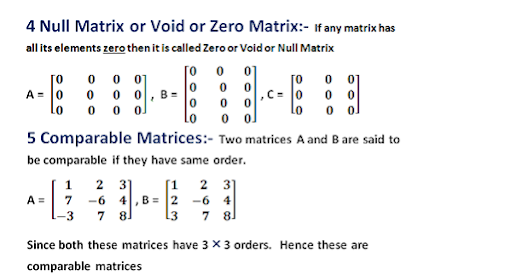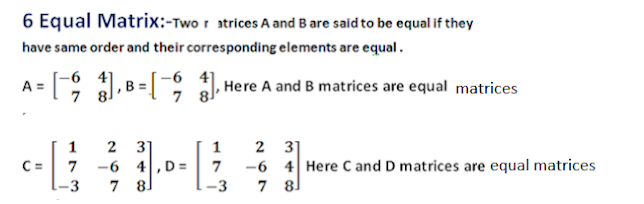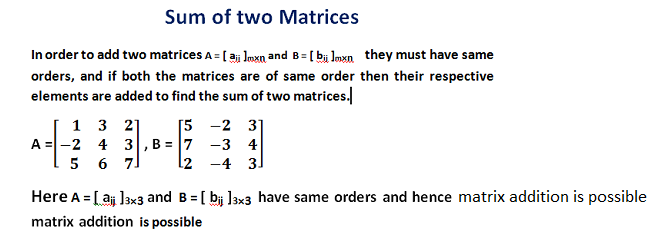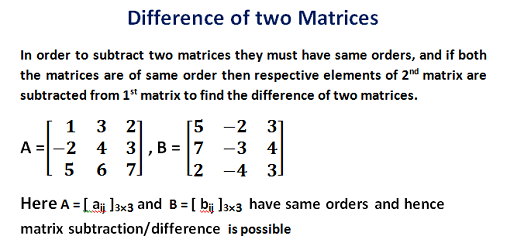Share: